JEE  >  Irodov Solutions: Radioactivity- 2

# Irodov Solutions: Radioactivity- 2 | I. E. Irodov Solutions for Physics Class 11 & Class 12 - JEE

 1 Crore+ students have signed up on EduRev. Have you?

Q.231. A radionuclide A1 goes through the transformation chain A→ A2 → A (stable) with respective decay constants λ1 and λ2. Assuming that at the initial moment the preparation contained only the radionuclide A1 equal in quantity to N10  nuclei, find the equation describing accumulation of the stable isotope A3.

Ans. Here we have the equations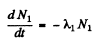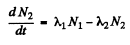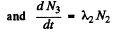From problem 229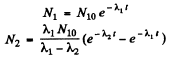Then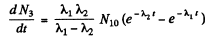or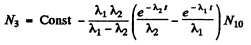since N3 = 0 initially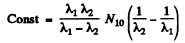So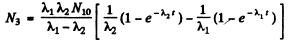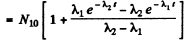Q.232. A Bi21° radionuclide decays via the chain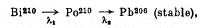where the decay constants are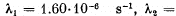5.80.10-8  s-1. Calculate alpha- and beta-activities of the Bi210 preparation of mass 1.00 mg a month after its manufacture.

Ans We have the chain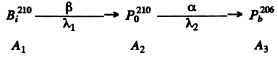of the previous problem initially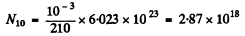A month after preparation

N1 = 4.54 x 1016

N2 = 2.52 x 1018

using the results of the previous problem.

Then

Aβ = λ1N1 = 0.725 x 1011 dis/sec

Aα = λ2N2 = 1.46 x 1011 dis/sec

Q.233. (a) What isotope is produced from the alpha-radioactive Ra228  as a result of five alpha-disintegrations and four β-disintegrations?
(b) How many alpha- and β-decays does U 238  experience before turning finally into the stable Pb206  isotope?

Ans.  (a) Ra has Z - 88, A - 226 After 3 a emission and 4 p (electron) emission

A - 206
Z - 88 + 4-5x2 - 82

The product is 82pb206

(b) We require

- ΔZ = 10 = 2 n - m

- ΔA = 32 = ft x 4

Here n = no. of α emissions

m = no. of β emissions

Thus n = 8, m = 6

Q.234. A stationary Pb200 nucleus emits an alpha-particle with kinetic energy Tα = 5.77 MeV. Find the recoil velocity of a daughter nucleus. What fraction of the total energy liberated in this decay is accounted for by the recoil energy of the daughter nucleus?

Ans. The momentum of the α-particle is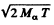This is also the recoil m om entum o f the daughter nuclear in opposite direction. The recoil velocity of the daughter ntideus is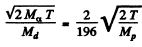= 3.39x105 m/S

The eneigy of the daughter nucleus is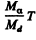and this represents a fraction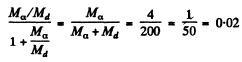of total eneigy. Here Md is the mass of the daughter nudeus.

Q.235. Find the amount of heat generated by 1.00 mg of a Po210 preparation during the mean lifetime period of these nuclei if the emitted alpha-particles are known to possess the kinetic energy 5.3 MeV and practically all daughter nuclei are formed directly in the ground state.

Ans. The number of nuclei initially present is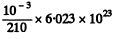In the mean life time of these nuclei the number decaying is the fraction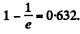Thus the eneigy released is 2.87 x 1018 x 0.632 x 5.3 x 1.602 x 10-13 J = 1.54MJ

Q.236. The alpha-decay of Po210 nuclei (in the ground state) is accompanied by emission of two groups of alpha-particles with kinetic energies 5.30 and 4.50 MeV. Following the emission of these particles the daughter nuclei are found in the ground and excited states. Find the energy of gamma-quanta emitted by the excited nuclei.

Ans. We neglect all recoil effects. Then the following diagram gives the eneigy of the gamma ray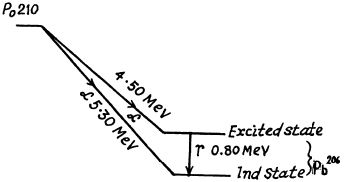Q.237. The mean path length of alpha-particles in air under standard conditions is defined by the formula R = 0.98.10-27  v3o cm, where v0 (cm/s) is the initial velocity of an alpha-particle. Using this formula, find for an alpha-particle with initial kinetic energy 7.0 MeV:
(a) its mean path length;
(b) the average number of ion pairs formed by the given alphaparticle over the whole path R as well as over its first half, assuming the ion pair formation energy to be equal to 34 eV.

Ans.  (a) For an alpha particle with initial K.E. 7.0 MeV, the initial velocity is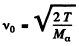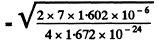= 1.83 x 109an/sec

Thus            R = 6.02an

(b) Over the whole path the number of ion pairs is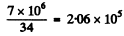Over the first half of the path We write the formula for the mean path as RαE1/2 where E is the initial energy. Thus if the energy of the a-particle after traversing the first half of the path is Ethen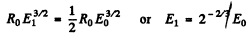Hence number of ion pairs formed in the first half of the path length is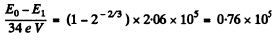Q.238. Find the energy Q liberated in β-- and β+-decays and in K-capture if the masses of the parent atom MP, the daughter atom Md and an electron m are known.

Ans.  In β- decay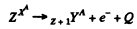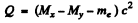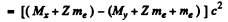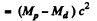since Mp, Md are the masses of the atoms. The binding energy of the electrons in ignored. In K capture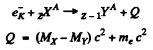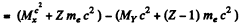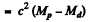In β+ decay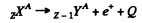Then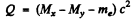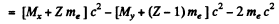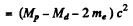Q.239. Taking the values of atomic masses from the tables, find the maximum kinetic energy of beta-particles emitted by Be10 nuclei and the corresponding kinetic energy of recoiling daughter nuclei formed directly in the ground state.

Ans.   The reaction is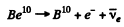For maximum K.E. of electrons we can put the energy of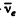to be zero. The atomic masses are

Be10 = 10.016711 amu

B10 = 10.016114 amu

So the K.E. of electrons is (see previous problem)

597 x 10-6 amu x c2 = 0.56 MeV

The momentum of electrons with this K.E. is 0.941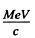and the recoil energy of the daughter is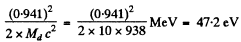Q.240. Evaluate the amount of heat produced during a day by a β--active Na24  preparation of mass m = 1.0 mg. The beta-particles are assumed to possess an average kinetic energy equal to 1/3 of the highest possible energy of the given decay. The half-life of Na24  is T = 15 hours.

Ans. The masses are

Na24 = 24 - 0.00903 amu and Mg24 = 24 - 0.01496 amu

The reaction is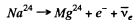The maximum K.E. of electrons is

0.00593 x 93 MeV = 5.52 MeV

Average K.E. according to the problem is then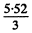= 1.84 MeV

The initial number of Na24 is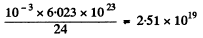The fraction decaying in a day is

1 - (2) - 24/15 = 0.67

Hence the heat produced in a day is

0.67 x 2.51 x 1019 x 1.84 x 1.602 x 10-13 Joul = 4.95MJ

Q.241. Taking the values of atomic masses from the tables, calculate the kinetic energies of a positron and a neutrino emitted by C11 nucleus for the case when the daughter nucleus does not recoil.

Ans.  We assume that the parent nucleus is at rest Then since the daughter nucleus does not recoil, we have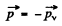i.e. positron & v mometum are equal and opposite. On the other hand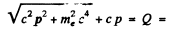total energy released. (Here we have used the fact that energy

of the neutrino is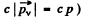Now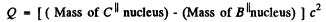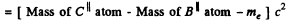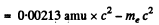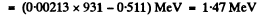Then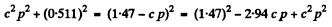Thus c p = 0.646 M eV = energy of neutrino

Also K.E. of electron = 1.47 - 0.646 - 0.511 = 0.313 MeV

Q.242. Find the kinetic energy of the recoil nucleus in the positronic decay of a Nn nucleus for the case when the energy of positrons is maximum.

Ans. The K.E. of the positron is maximum when the energy of neutrino is zero. Since the recoil energy of the nucleus is quite small, it can be calculated by successive approximation.
The reaction is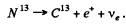The maximum energy available to the positron (including its rest energy) is

c2 (Mass of N13 nucleus - Mass of C13 nucleus)

= c2 (Mass of N13 atom - Mass of C13 atom - me)

= 0.00239 c2 - mec2

= (0.00239 x 931 - 0.511) MeV

= 1.71 MeV

The momentum corresponding to this energy is 1.636 MeV/c .

The recoil energy of the nucleus is then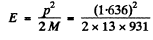= 111 eV = 0.111 keV

on using Mc2 - 13 x 931 MeV

Q.243. From the tables of atomic masses determine the velocity of a nucleus appearing as a result of K-capture in a Bel atom provided the daughter nucleus turns out to be in the ground state.

Ans. The process is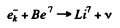The energy available in the process is

Q = c2 (Mass of Be7 atom - Mass of Li-7 atom)

= 0.00092 x 931 MeV = 0.86 MeV

The momentum of a K electron is negligible. So in the rest frame of the Be7 atom, most of the energy is taken by neutrino whose momentum is very nearly 0.86MeV/c The momentum of the recoiling nucleus is equal and opposite. The velocity of recoil is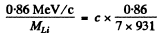= 3.96 x106 cm/s

Q.244. Passing down to the ground state, excited Ag109 in nuclei emit either gamma quanta with energy 87 keV or K conversion electrons whose binding energy is 26 keV. Find the velocity of these electrons.

Ans.  In internal conversion, the total energy is used to knock out K electrons. The KE. of these electrons is energy available-B.E. of K electrons

= (87 - 26) = 61 keV

The total energy including rest mass of electrons is 0.511 + 0.061 = 0.572 MeV

The momentum corresponding to this total energy is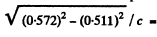0257 MeV/c.

The velocity is then =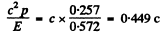Q.245. A free stationary Ir191 nucleus with excitation energy E = 129 keV passes to the ground state, emitting a gamma quantum. Calculate the fractional change of gamma quanta energy due to recoil of the nucleus.

Ans. With recoil neglected, the y-quantram will have 129 keV eneigy. To a first approximation, its momentrum will be 129 keV/c and the energy of recoil will be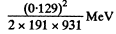= 4.18 x 10- 8 MeV

In the next approximation we therefore write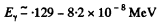Therefore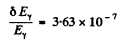Q.246. What must be the relative velocity of a source and an absorber consisting of free Ir191 nuclei to observe the maximum absorption of gamma quanta with energy ε = 129 keV?

Ans. For maximum (resonant) absorption, the absorbing nucleus must be moving with enough speed to cancel the momentum of the oncoming photon and have just right eneigy (ε = 129 keV) available for transition to the excited state.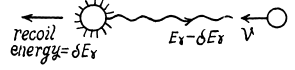Since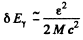and momentum of the photon is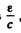these condition can be satisfied if the velocity of the nucleus is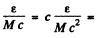218m/s = 0.218km/s

Q.247. A source of gamma quanta is placed at a height h = 20m above an absorber. With what velocity should the source be displaced upward to counterbalance completely the gravitational variation of gamma quanta energy due to the Earth's gravity at the point where the absorber is located?

Ans. Because of the gravitational shift the frequency of the gamma ray at the location of the absorber is increased by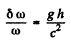For this to be compensated by the Doppler shift (assuming that resonant absorption is possible in the absence of gravitational field) we must have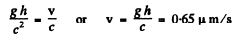Q.248. What is the minimum height to which a gamma quanta source containing excited Zn67  nuclei has to be raised for the gravitational displacement of the Mossbauer line to exceed the line width itself, when registered on the Earth's surface? The registered gamma quanta are known to have an energy ε = 93 keV and appear on transition of Zn67 nuclei to the ground state, and the mean lifetime of the excited state is ζ = 14μS.

Ans. The natural life time is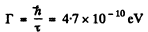Thus the condition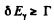implies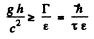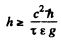= 4.64 metre

(h here is height of the place, not planck’s constant.)

The document Irodov Solutions: Radioactivity- 2 | I. E. Irodov Solutions for Physics Class 11 & Class 12 - JEE is a part of the JEE Course I. E. Irodov Solutions for Physics Class 11 & Class 12.
All you need of JEE at this link: JEE

111 docs

## I. E. Irodov Solutions for Physics Class 11 & Class 12

111 docs

Track your progress, build streaks, highlight & save important lessons and more!

,

,

,

,

,

,

,

,

,

,

,

,

,

,

,

,

,

,

,

,

,

;